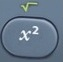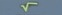# Square Root

Square Root =  Number² = √ Number × Itself

Basics
Square Root = Number² = √Number × Itself

Square Root of 9 = 9 =  = √3 × 3 = 3
Square Root of 144 = √144  = √12² = √12 × 12 = 12
Square Root of 412.09 = 412.09  = 20.3² = √20.3 × 20.3 = 20.3

The root sign √   is also known as the radical sign.

When the root sign    is immediately adjacent to a number or letter, an invisible multiplication sign is in force.
53  =  5 × √3
a3  =  a × √3is the square root command.activatesabove.

It is possible that questions involving simplifying square roots will be in the
calculator-prohibited section of the test.  In that case, it is helpful to know your multiplication and division tables.

When simplifying square roots without a calculator, a variation of factoring is used to extract from pairs of numbers under the root sign.

The numbers 2 and 3 are often useful in factoring.

Formulas (NOT provided by Mathematics Formula Sheet)

Square Root = Number² = √Number × Itself

NumberA × √NumberB = √NumberA × NumberB

Question
What is 16 ?

4

Calculator Click What You See Commentblinker clears screen16 16 Square Root4 Answer
###### Square Root

Question

What is 5097.96 ?

71.4

Calculator Click What You See Commentblinker clears screen5097.96 5097.96 Square Root71.4 Answer
###### Square Root

Question

Using the calculator, what is the simplified version of 180 ?

65

Calculator Click What You See Commentblinker clears screen180 180 Square Root65 Answer
###### Square Root

Question

Without using the calculator, what is the simplified version of 180 ?

65

When simplifying square roots without a calculator, a variation of factoring is used to extract from pairs of numbers under the root sign:

Factoring (Variation):
180               ÷         2              =          90
Multiple                 Factor

90               ÷           2               =         45
Multiple                 Factor

45               ÷           3               =         15
Multiple                 Factor

15               ÷           3               =         5
Multiple                 Factor

5               ÷            5               =          1
Multiple                Factor

180  =  2 × 2 × 3 × 3 × 5  =  2 × 3√5  =  6√5
2 is extracted from a pair of 2’s under the root sign;
3 is extracted from a pair of 3’s under the root sign;
an unpaired 5 is “left behind” under the root sign.

Question
Using the calculator, what is the simplified version of √6  × √12 ?

62

Calculator Click What You See Commentblinker clears screen×12 6  × √126√2 Answer
###### Square Root

Question
Without using the calculator, what is the simplified version of √6 × √12 ?

62

NumberA × √NumberB = √NumberA × NumberB
6 × √12 = √6×12 = √72 = 62

When simplifying square roots without a calculator, a variation of factoring is used to extract from pairs of numbers under the root sign:

Factoring (Variation):
72               ÷         2              =          36
Multiple               Factor

36               ÷         2               =         18
Multiple                Factor

18               ÷          2               =         9
Multiple                Factor

9               ÷           3               =         3
Multiple               Factor

3               ÷           3               =         1
Multiple               Factor

72  =  2 × 2 × 2 × 3 × 3 =  2 × 32  =  62
2 is extracted from a pair of 2’s under the root sign;
3 is extracted from a pair of 3’s under the root sign;
an unpaired 2 is “left behind” under the root sign.

Question

What is 2 in decimal terms?

1.414213562

See Toggle.
2  ⇔ 1.414213562

Calculator Click What You See Commentblinker clears screen2 221.414213562 Answer
(Toggle)
###### Square Root

Question

What is 62 in decimal terms?

8.485281374

See Toggle.
6√2  ⇔ 8.485281374

Calculator Click What You See Commentblinker clears screen
6 ×2 6*√26√28.485281374 Answer
(Toggle)
###### Square Root

Question
With reference to the question above, what is 62 in decimal terms rounded to the nearest hundredth?

8.49

See Rounding.
8.485281374  8.49

Practice – Questions
1.  What is 49 ?

2.  What is 2766.76 ?

3.  Using the calculator, what is the simplified version of 108 ?

4.  Without using the calculator, what is the simplified version of 108 ?

5.  Using the calculator, what is the simplified version of √5 × √15  ?

6.  Without using the calculator, what is the simplified version of √5 × √15  ?

7. Without using the calculator, what is the simplified version of 48 ?

8. Without using the calculator, what is the simplified version of √6 × √14  ?

9.  What is 43 in decimal terms?

10.  With reference to Question 9, what is 43 in decimal terms rounded to the nearest tenth?

1.  7

2.  52.6

3.  63

4.
Factoring (Variation):
108               ÷         2              =          54
Multiple                 Factor

54               ÷           2               =         27
Multiple                 Factor

27               ÷           3               =         9
Multiple                 Factor

9               ÷            3               =         3
Multiple                 Factor

3               ÷            3               =          1
Multiple                Factor

108  =  2 × 2 × 3 × 3 × 3  =  2 × 3√3  =  6√3
2 is extracted from a pair of 2’s under the root sign;
3 is extracted from a pair of 3’s under the root sign;
an unpaired 3 is “left behind” under the root sign.

5.  53

6.
NumberA × √NumberB = √NumberA × NumberB
5 × √15 = √5×15 = √75 = 53

Factoring (Variation):
75               ÷           5              =          15
Multiple                 Factor

15               ÷           5               =         3
Multiple                 Factor

3              ÷              3               =         1
Multiple                 Factor

75  =  5 × 5 × 3  =  5√3
5 is extracted from a pair of 5’s under the root sign;
an unpaired 3 is “left behind” under the root sign.

7.  43

8.  221

9.  6.92820323

10.  6.9